## Grade 9 Algebra Word Problems

Related Pages Grade 8 Algebra Word Problems Algebra Word Problems Solving Equations More Algebra Lessons

These lessons cover grade 9 algebra word problems involving age, distance, rate, time and coins with examples and step-by-step solutions. It includes various examples and solutions for algebra word problems that you will commonly encounter in 9th grade.## Age Word Problems

Age Problems with two unknowns or variables

Example: Taylor is five times as old as Spenser. The sum of their ages is eighteen. How old are Taylor and Spencer?

Solution: Let x represent Spenser’s age Therefore, Taylor’s age is 5x x + 5x = 18 6x = 18 x = 3 Therefore, Spenser is 3 years old and Taylor is 15 years old.

Grade 9 Algebra Word Problems - Age

Example 1: A mother is three times as old as her daughter. Six years ago, the mother’s age was six tines that of her daughter. How old are they now?

Solution: Let x represent the daughter’s age. Therefore, 3x is the mother’s age. 6(x - 6) = 3x - 6 6x - 6 = 3x - 6 3x = 30 x = 10 Therefore, the daughter’s is 10 years old and the mother is 30 years old.

Example 2: A father is now three times as old as his son. Eight years ago, the father was five times as old as his son. How old are they now?

## Rate, Distance, Time Word Problems

Grade 9 Algebra Word Problems - Rate, Distance, Time

Example: A bus leaves the terminal and averages 40 km/hr. One hour late, a second bus leaves the same terminal and averages 50 km/hr. In how many hours will the second bus overtake the first?

Grade 9 Rate, Distance, Time Word Problems

Example 1: One motorist travels 5 km.hr faster than another. They leave from the same place and travel in opposite directions. What is the rate of each if they are 195 km apart after 3 hours?

Example 2: A pilot flew from airport A to airport B at a rate of 100 km/hr and flew back from airport B to airport A at 120 km/hr. The total time it took was 11 hours. How far is it from airport A to airport B?

## Coin Word Problems

Grade 9 Algebra Word Problems - Coins

Example: A coin collection amounting to \$25 consists of nickels and dimes. There are 3 times as many nickels and dimes. There are 3 times as many nickels as dimes. How many coins of each kind are there?

Solution: Let x = number of dimes 3x = number of nickels 10x + 5(3x) = 2500 25x = 2500 x = 100 Therefore, there are 100 dimes and 300 nickels.

Grade 9 Coin Algebra Word Problems

Example: Mr. Rogers has \$4.62. He has 3 times as many dimes as nickels and 6 more pennies than dimes. How many coins of each kind does he have?

Coin Algebra Word Problems - Grade 9

Example: Bob has in his pocket a number of pennies, 5 times as many nickels as pennies and 5 more quarters than pennies. The coins amount to \$2.27. Find the number of each kind of coin.• Kindergarten
• Arts & Music
• English Language Arts
• World Language
• Social Studies - History
• Special Education
• Holidays / Seasonal
• Independent Work Packet
• Easel by TPT## Easel Activities## Easel Assessments

All formats, resource types, all resource types, 9th grade word problems worksheets free.

• Rating Count
• Price (Ascending)
• Price (Descending)
• Most Recent## Bundle Maya Aztec Inca Math Activity Symmetry Hispanic Heritage Month## Mexico Math Activity Mexico Symmetry Frida Kahlo Cactus Hispanic Heritage Month## Math Activities Lab Bundle 6th Grade - Math Intervention - Sub Plans## Personal Finance Unit - PBL Simulation - Print and Google™ Drive## HIGH SCHOOL WRITING WORKSHOP. Punctuation & Writing Practice. ELA Worksheets## 50 Academic Vocabulary Words for ELA - Lessons and Digital Flashcards## PRINTABLE Murder Mystery Escape, Middle, High School Team-building Escape## #1 Science Curriculum Bundle | Physical, Earth, Space & Biology Life Science## SYSTEMS OF EQUATIONS REAL-LIFE PROBLEMS## Translating word problems into algebraic expressions• Word Document File## The Wizard - Math Challenge Problem## Data Analysis Worksheet - Reading & Analyzing Stem and Leaf Plots## Mathistory MLK Jr. Day## Venn Diagrams from Word Problems -- Problem solving## Distance Rate Time System Organizer, Explication and Summary## Surface Area of a Cylinder w/ Answer Key## Test Corrections/Error Analysis## Interactive Notebook Integer Application Practice## Seven Tips for Math Success & Graphic Organizer {FREEBIE}## St. Patrick's Day Math Activities## Enduring Issues Vocabulary List## Hole Punch- Pythagorean Theorem## Cut and Paste Linear Word Problems## Area Perimeter Australia Farmer Metric## Sinusoidal Functions- Word Problems## Math 1 Spiral Reviews Set #2: FREEBIE## Patterns with Pinecones## Probability - 100 Word Problems with detailed solutions and insights## Ground Hog Day Quadratic Equation Story Problems## Proportion to Powers of a Variable PPT and Worksheets## Angles of Elevation and Depression Star Worksheet## Middle School Math Vocabulary Checklist (8th Grade)• We're Hiring
• Help & FAQ
• Student Privacy
• + ACCUPLACER Mathematics
• + ACT Mathematics
• + AFOQT Mathematics
• + ALEKS Tests
• + ASVAB Mathematics
• + ATI TEAS Math Tests
• + Common Core Math
• + DAT Math Tests
• + FSA Tests
• + FTCE Math
• + GED Mathematics
• + Georgia Milestones Assessment
• + GRE Quantitative Reasoning
• + HiSET Math Exam
• + HSPT Math
• + ISEE Mathematics
• + PARCC Tests
• + Praxis Math
• + PSAT Math Tests
• + PSSA Tests
• + SAT Math Tests
• + SBAC Tests
• + SIFT Math
• + SSAT Math Tests
• + STAAR Tests
• + TABE Tests
• + TASC Math
• + TSI Mathematics
• + ACT Math Worksheets
• + Accuplacer Math Worksheets
• + AFOQT Math Worksheets
• + ALEKS Math Worksheets
• + ASVAB Math Worksheets
• + ATI TEAS 6 Math Worksheets
• + FTCE General Math Worksheets
• + GED Math Worksheets
• + 3rd Grade Mathematics Worksheets
• + 4th Grade Mathematics Worksheets
• + 5th Grade Mathematics Worksheets
• + 6th Grade Math Worksheets
• + 7th Grade Mathematics Worksheets
• + 8th Grade Mathematics Worksheets
• + 9th Grade Math Worksheets
• + HiSET Math Worksheets
• + HSPT Math Worksheets
• + ISEE Middle-Level Math Worksheets
• + PERT Math Worksheets
• + Praxis Math Worksheets
• + PSAT Math Worksheets
• + SAT Math Worksheets
• + SIFT Math Worksheets
• + SSAT Middle Level Math Worksheets
• + 7th Grade STAAR Math Worksheets
• + 8th Grade STAAR Math Worksheets
• + THEA Math Worksheets
• + TABE Math Worksheets
• + TASC Math Worksheets
• + TSI Math Worksheets
• + AFOQT Math Course
• + ALEKS Math Course
• + ASVAB Math Course
• + ATI TEAS 6 Math Course
• + CHSPE Math Course
• + FTCE General Knowledge Course
• + GED Math Course
• + HiSET Math Course
• + HSPT Math Course
• + ISEE Upper Level Math Course
• + SHSAT Math Course
• + SSAT Upper-Level Math Course
• + PERT Math Course
• + Praxis Core Math Course
• + SIFT Math Course
• + 8th Grade STAAR Math Course
• + TABE Math Course
• + TASC Math Course
• + TSI Math Course
• + Number Properties Puzzles
• + Algebra Puzzles
• + Geometry Puzzles
• + Intelligent Math Puzzles
• + Ratio, Proportion & Percentages Puzzles
• + Other Math Puzzles

## 9th Grade Math Worksheets: FREE & Printable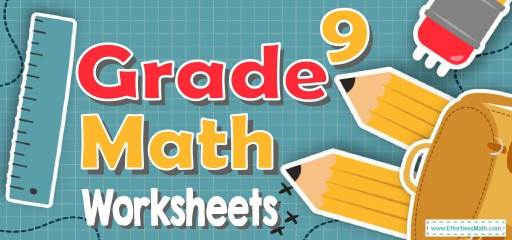Want to measure your 9th-grade student’s knowledge of Math concepts and assess their exam readiness? If so, then look no further.

Here is a comprehensive collection of FREE printable 9th-grade Math worksheets that would help students in 9th Grade Math preparation and practice.

Hope you enjoy it!

IMPORTANT: COPYRIGHT TERMS: Worksheets may not be uploaded to the internet, including classroom/personal websites or network drives. You can download the worksheets and print as many as you need. You can distribute the printed copies to your students, teachers, tutors, and friends.

You Do NOT have permission to send these worksheets to anyone in any way (via email, text messages, or other ways). They MUST download the worksheets themselves. You can send the address of this page to your students, tutors, friends, etc.

## Related Topics

The Absolute Best Book to Ace 9th Grade Math

## Algebra I for Beginners The Ultimate Step by Step Guide to Acing Algebra I

• Translate Phrases into an Algebraic Statement
• Order of Operations
• Properties of Numbers
• The Distributive Property
• Scientific Notation

## Expressions and Equations

• Simplifying Variable Expressions
• One–Step Equations
• Multi–Step Equations
• Finding Midpoint
• Finding Distance of Two Points
• Absolute Value Equations

## Linear Functions

• Finding Slope
• Graphing Lines Using Line Equation
• Writing Linear Equations
• Graphing Linear Inequalities
• Slope and Rate of Change
• Find the Slope x–intercept and y–intercept
• Write an Equation from a Graph
• Slope –intercept Form and Point –slope Form
• Equations of Horizontal and Vertical Lines
• Equation of Parallel or Perpendicular Lines
• Graphing Absolute Value Equations
• Greatest Common Factor
• Least Common Multiple
• GCF of Monomials
• Factoring by Grouping

## Inequalities and System of Equations

• One–Step Inequalities
• Two–Step Linear Inequalities
• Solve Compound Inequalities
• Solve Absolute Value Inequalities
• Systems of Equations
• Systems of Equations Word Problems

## Monomials Operations

• Multiply and Divide Monomials
• Solve a Quadratic Equation by Factoring
• Quadratic Formula and the Discriminant

## Polynomial Operations

• Simplifying Polynomials
• Multiplying a Polynomial and a Monomial
• Multiply Two Polynomials
• Multiply Two Binomials
• Operations with Polynomials
• Factor Polynomials
• Factoring Special Case Polynomials

• Simplifying Radical Expressions Involving Fractions
• Domain and Range of Radical Functions

## Rational Expressions

• Simplifying and Graphing Rational Expressions
• Adding and Subtracting Rational Expressions
• Multiplying and Dividing Rational Expressions
• Solving Rational Equations and Complex Fractions## Statistics and Probabilities

• Probability Problems
• Combinations and Permutations

## Statistics and Probabilities

9th grade math practice questions, fractions and decimals, real numbers and integers, proportions and ratios, algebraic expressions, equations and inequalities, polynomials, exponents and radicals, solid figures.

Looking for the best resource to help you succeed on the Algebra I test?

The Best Books to Ace Algebra

## Algebra II for Beginners The Ultimate Step by Step Guide to Acing Algebra II

Pre-algebra for beginners the ultimate step by step guide to preparing for the pre-algebra test, pre-algebra tutor everything you need to help achieve an excellent score.

by: Reza about 3 years ago (category: Blog , Free Math Worksheets )

Reza is an experienced Math instructor and a test-prep expert who has been tutoring students since 2008. He has helped many students raise their standardized test scores--and attend the colleges of their dreams. He works with students individually and in group settings, he tutors both live and online Math courses and the Math portion of standardized tests. He provides an individualized custom learning plan and the personalized attention that makes a difference in how students view math.

More math articles.

• Subtraction of Four-Digit Numbers
• Top 10 7th Grade MCAS Math Practice Questions
• Everything You Need to Know to Choose the Right Laptop
• Top 10 Tips to Improve Your Low SAT Math Score
• How to Rearranging a Multi-Variable Equation
• The Best Teacher Desks in 2023
• Best Office Chairs for Online Teaching
• The Ultimate 4th Grade STAAR Math Course (+FREE Worksheets)
• The Ultimate ISTEP Algebra 1 Course (+FREE Worksheets)
• A Comprehensive Collection of Free GED Math Practice Tests

## What people say about "9th Grade Math Worksheets: FREE & Printable - Effortless Math: We Help Students Learn to LOVE Mathematics"?

No one replied yet.

## The Ultimate Algebra Bundle From Pre-Algebra to Algebra II

• ATI TEAS 6 Math
• ISEE Upper Level Math
• SSAT Upper-Level Math
• Praxis Core Math

Limited time only!

Save Over 49 %

It was \$69.99 now it is \$35.99

## Login and use all of our services.

Effortless Math services are waiting for you. login faster!

## Register Fast!

• Math Worksheets
• Math Courses
• Math Topics
• Math Puzzles
• Math eBooks
• GED Math Books
• HiSET Math Books
• ACT Math Books
• ISEE Math Books
• ACCUPLACER Books
• Apple Store

Effortless Math provides unofficial test prep products for a variety of tests and exams. All trademarks are property of their respective trademark owners.

• Bulk Orders
• Refund Policy

## Free Printable Math Worksheets for 9th Grade

Math just got more exciting for Grade 9 students! Discover a vast collection of free printable math worksheets by Quizizz, tailored to enhance learning and understanding. Dive into a world of fun and interactive problem-solving activities that will transform your classroom experience.## Explore worksheets by Math topics

• Expressions and Equations
• Linear Functions
• Inequalities and System of Equations
• Monomials Operations
• Polynomial Operations
• Rational Expressions
• Statistics and Probabilities
• arithmetic and number theory
• probability and statistics
• trigonometry## Explore Math worksheets by grade

• kindergarten
• worksheets  /

## Explore printable Math worksheets for 9th Grade

Math worksheets for Grade 9 are an essential resource for teachers to help their students master the various mathematical concepts and skills required at this level. These worksheets cover a wide range of topics, including algebra, geometry, trigonometry, and statistics, providing ample practice for students to build a strong foundation in mathematics. Teachers can use these worksheets to supplement their lesson plans, assign as homework, or provide additional practice for struggling students. With a variety of question types and difficulty levels, Grade 9 math worksheets cater to the diverse learning needs of students, ensuring that they are well-prepared for the challenges of high school mathematics.

Quizizz is an excellent platform for teachers to access a vast collection of Math worksheets for Grade 9, along with other valuable resources such as quizzes, interactive lessons, and games. This platform allows teachers to easily create and customize their own worksheets, tailoring them to the specific needs of their students. In addition to worksheets, Quizizz offers engaging and interactive quizzes that can be used for formative assessments or as a fun way to review and reinforce mathematical concepts. Teachers can also take advantage of the platform's analytics and reporting features to track student progress and identify areas where additional support may be needed. By incorporating Quizizz into their teaching toolkit, educators can provide their Grade 9 students with a comprehensive and engaging learning experience that fosters mathematical proficiency and confidence.

## Math Word Problems

Welcome to the math word problems worksheets page at Math-Drills.com! On this page, you will find Math word and story problems worksheets with single- and multi-step solutions on a variety of math topics including addition, multiplication, subtraction, division and other math topics. It is usually a good idea to ensure students already have a strategy or two in place to complete the math operations involved in a particular question. For example, students may need a way to figure out what 7 × 8 is or have previously memorized the answer before you give them a word problem that involves finding the answer to 7 × 8.

There are a number of strategies used in solving math word problems; if you don't have a favorite, try the Math-Drills.com problem-solving strategy:

• Question : Understand what the question is asking. What operation or operations do you need to use to solve this question? Ask for help to understand the question if you can't do it on your own.
• Estimate : Use an estimation strategy, so you can check your answer for reasonableness in the evaluate step. Try underestimating and overestimating, so you know what range the answer is supposed to be in. Be flexible in rounding numbers if it will make your estimate easier.
• Strategize : Choose a strategy to solve the problem. Will you use mental math, manipulatives, or pencil and paper? Use a strategy that works for you. Save the calculator until the evaluate stage.
• Calculate : Use your strategy to solve the problem.
• Evaluate : Compare your answer to your estimate. If you under and overestimated, is the answer in the correct range. If you rounded up or down, does the answer make sense (e.g. is it a little less or a little more than the estimate). Also check with a calculator.

## Most Popular Math Word Problems this Week## Various Word Problems

Various word problems for students who have mastered basic arithmetic and need a further challenge.## Subtraction word problems## Multiplication word problems## Division word problems## Multi-Step word problems• Kindergarten
• Learning numbers
• Comparing numbers
• Place Value
• Roman numerals
• Subtraction
• Multiplication
• Order of operations
• Drills & practice
• Measurement
• Factoring & prime factors
• Proportions
• Shape & geometry
• Data & graphing
• Word problems
• Children's stories
• Leveled Stories
• Context clues
• Cause & effect
• Compare & contrast
• Fact vs. fiction
• Fact vs. opinion
• Main idea & details
• Story elements
• Conclusions & inferences
• Sounds & phonics
• Words & vocabulary
• Early writing
• Numbers & counting
• Simple math
• Social skills
• Other activities
• Dolch sight words
• Fry sight words
• Multiple meaning words
• Prefixes & suffixes
• Vocabulary cards
• Other parts of speech
• Punctuation
• Capitalization
• Cursive alphabet
• Cursive letters
• Cursive letter joins
• Cursive words
• Cursive sentences
• Cursive passages
• Grammar & Writing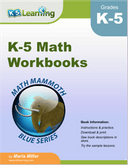## Free Math Worksheets

Printable math worksheets from k5 learning.

Our  free math worksheets  cover the full range of elementary school math skills from numbers and counting through fractions, decimals, word problems and more. All worksheets are printable files with answers on the 2nd page.

Math worksheets by topic:.Sample Math Worksheet

What is K5?

K5 Learning offers free worksheets , flashcards  and inexpensive  workbooks  for kids in kindergarten to grade 5. Become a member  to access additional content and skip ads.Our members helped us give away millions of worksheets last year.

We provide free educational materials to parents and teachers in over 100 countries. If you can, please consider purchasing a membership (\$24/year) to support our efforts.

Members skip ads and access exclusive features.This content is available to members only.• Kindergarten
• Number charts
• Skip Counting
• Place Value
• Number Lines
• Subtraction
• Multiplication
• Word Problems
• Comparing Numbers
• Ordering Numbers
• Odd and Even
• Prime and Composite
• Roman Numerals
• Ordinal Numbers
• In and Out Boxes
• Number System Conversions
• More Number Sense Worksheets
• Size Comparison
• Measuring Length
• Metric Unit Conversion
• Customary Unit Conversion
• Temperature
• More Measurement Worksheets
• Writing Checks
• Profit and Loss
• Simple Interest
• Compound Interest
• Tally Marks
• Mean, Median, Mode, Range
• Mean Absolute Deviation
• Stem-and-leaf Plot
• Box-and-whisker Plot
• Permutation and Combination
• Probability
• Venn Diagram
• More Statistics Worksheets
• Shapes - 2D
• Shapes - 3D
• Lines, Rays and Line Segments
• Points, Lines and Planes
• Transformation
• Ordered Pairs
• Midpoint Formula
• Distance Formula
• Parallel, Perpendicular and Intersecting Lines
• Scale Factor
• Surface Area
• Pythagorean Theorem
• More Geometry Worksheets
• Converting between Fractions and Decimals
• Significant Figures
• Convert between Fractions, Decimals, and Percents
• Proportions
• Direct and Inverse Variation
• Order of Operations
• Squaring Numbers
• Square Roots
• Scientific Notations
• Speed, Distance, and Time
• Absolute Value
• More Pre-Algebra Worksheets
• Translating Algebraic Phrases
• Evaluating Algebraic Expressions
• Simplifying Algebraic Expressions
• Algebraic Identities
• Systems of Equations
• Polynomials
• Inequalities
• Sequence and Series
• Complex Numbers
• More Algebra Worksheets
• Trigonometry
• Math Workbooks
• English Language Arts
• Summer Review Packets
• Social Studies
• Holidays and Events
• Worksheets >
• Number Sense >

## Math Word Problem Worksheets

Read, explore, and solve over 1000 math word problems based on addition, subtraction, multiplication, division, fraction, decimal, ratio and more. These word problems help children hone their reading and analytical skills; understand the real-life application of math operations and other math topics. Print our exclusive colorful theme-based worksheets for a fun-filled teaching experience! Use the answer key provided below each worksheet to assist children in verifying their solutions.

## List of Word Problem Worksheets

Explore the word problem worksheets in detail.

Have 'total' fun by adding up a wide range of addends displayed in these worksheets! Simple real-life scenarios form the basis of these addition word problem worksheets.

Subtraction Word Problems

Learning can be a huge 'take away'! Find the difference between the numbers provided in each subtraction word problem. Large number subtraction up to six-digits can also be found here.

Bring on 'A' game with our addition and subtraction word problems! Read, analyze, and solve real-life scenarios based on adding and subtracting numbers as required.

Multiplication Word Problems

Get 'product'ive with over 100 highly engaging multiplication word problems! Find the product and use the answer key to verify your solution. Free worksheets are also available.

Division Word Problems

"Divide and conquer" this huge collection of division word problems. Exclusive worksheets are available for the division problem leaving no remainder and with the remainder.

Fraction Word Problems

Perform various mathematical operations to solve the umpteen number of word problems based on like and unlike fractions, proper and improper fractions, and mixed numbers.

Decimal Word Problems

Let's get to the 'point'! Add, subtract, multiply, and divide to solve these decimal word problems. A wide selection of printable worksheets is available in this section. Use the answer key to verify your answers.

Ratio Word problems

Double up your success ratio with these sets of word problems, which cover a multitude of topics like express in the ratio, reducing the ratio, part-to-part ratio, part-to-whole ratio and more.

Venn Diagram Word Problems - Two Sets

Help your children improve their data analysis skills with these well-researched Venn diagram word problem worksheets. Find the union, intersection, complement and difference of two sets.

Venn Diagram Word Problems - Three Sets

These Venn diagram word problems provide ample practice in real-life application of Venn diagram involving three sets. The worksheets containing the universal set are also included.

Equation Word Problems

The printable worksheets here feature exercises consisting of one-step, two-step and multi-step equation word problems involving fractions, decimals and integers. MCQs to test the knowledge acquired have also been included.

Sample Worksheets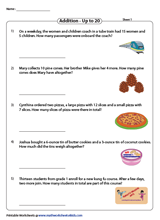Become a Member

Membership Information

What's New?

Printing Help

Testimonial## Free Math Worksheets — Over 100k free practice problems on Khan Academy

You’ve found something even better!

That’s because Khan Academy has over 100,000 free practice questions. And they’re even better than traditional math worksheets – more instantaneous, more interactive, and more fun!

Kindergarten, basic geometry, pre-algebra, algebra basics, high school geometry.

• Trigonometry

## Statistics and probability

High school statistics, ap®︎/college statistics, precalculus, differential calculus, integral calculus, ap®︎/college calculus ab, ap®︎/college calculus bc, multivariable calculus, differential equations, linear algebra.

• Place value (tens and hundreds)
• Addition and subtraction within 20
• Addition and subtraction within 100
• Addition and subtraction within 1000
• Measurement and data
• Counting and place value
• Measurement and geometry
• Place value
• Measurement, data, and geometry
• Add and subtract within 20
• Add and subtract within 100
• Add and subtract within 1,000
• Money and time
• Measurement
• Intro to multiplication
• 1-digit multiplication
• Intro to division
• Understand fractions
• Equivalent fractions and comparing fractions
• More with multiplication and division
• Arithmetic patterns and problem solving
• Represent and interpret data
• Multiply by 1-digit numbers
• Multiply by 2-digit numbers
• Factors, multiples and patterns
• Multiply fractions
• Understand decimals
• Plane figures
• Measuring angles
• Area and perimeter
• Units of measurement
• Decimal place value
• Subtract decimals
• Multi-digit multiplication and division
• Divide fractions
• Multiply decimals
• Divide decimals
• Powers of ten
• Coordinate plane
• Algebraic thinking
• Converting units of measure
• Properties of shapes
• Ratios, rates, & percentages
• Arithmetic operations
• Negative numbers
• Properties of numbers
• Variables & expressions
• Equations & inequalities introduction
• Data and statistics
• Negative numbers: addition and subtraction
• Negative numbers: multiplication and division
• Fractions, decimals, & percentages
• Rates & proportional relationships
• Expressions, equations, & inequalities
• Numbers and operations
• Solving equations with one unknown
• Linear equations and functions
• Systems of equations
• Geometric transformations
• Data and modeling
• Volume and surface area
• Pythagorean theorem
• Transformations, congruence, and similarity
• Arithmetic properties
• Factors and multiples
• Negative numbers and coordinate plane
• Ratios, rates, proportions
• Equations, expressions, and inequalities
• Exponents, radicals, and scientific notation
• Foundations
• Algebraic expressions
• Linear equations and inequalities
• Graphing lines and slope
• Expressions with exponents
• Equations and geometry
• Algebra foundations
• Solving equations & inequalities
• Working with units
• Linear equations & graphs
• Forms of linear equations
• Inequalities (systems & graphs)
• Absolute value & piecewise functions
• Exponential growth & decay
• Irrational numbers
• Performing transformations
• Transformation properties and proofs
• Right triangles & trigonometry
• Non-right triangles & trigonometry (Advanced)
• Analytic geometry
• Conic sections
• Solid geometry
• Polynomial arithmetic
• Complex numbers
• Polynomial factorization
• Polynomial division
• Polynomial graphs
• Exponential models
• Transformations of functions
• Rational functions
• Trigonometric functions
• Non-right triangles & trigonometry
• Trigonometric equations and identities
• Analyzing categorical data
• Displaying and comparing quantitative data
• Summarizing quantitative data
• Modeling data distributions
• Exploring bivariate numerical data
• Study design
• Probability
• Counting, permutations, and combinations
• Random variables
• Sampling distributions
• Confidence intervals
• Significance tests (hypothesis testing)
• Two-sample inference for the difference between groups
• Inference for categorical data (chi-square tests)
• Advanced regression (inference and transforming)
• Analysis of variance (ANOVA)
• Scatterplots
• Data distributions
• Two-way tables
• Binomial probability
• Normal distributions
• Displaying and describing quantitative data
• Inference comparing two groups or populations
• Chi-square tests for categorical data
• More on regression
• Prepare for the 2020 AP®︎ Statistics Exam
• AP®︎ Statistics Standards mappings
• Polynomials
• Composite functions
• Probability and combinatorics
• Limits and continuity
• Derivatives: definition and basic rules
• Derivatives: chain rule and other advanced topics
• Applications of derivatives
• Analyzing functions
• Parametric equations, polar coordinates, and vector-valued functions
• Applications of integrals
• Differentiation: definition and basic derivative rules
• Differentiation: composite, implicit, and inverse functions
• Contextual applications of differentiation
• Applying derivatives to analyze functions
• Integration and accumulation of change
• Applications of integration
• AP Calculus AB solved free response questions from past exams
• AP®︎ Calculus AB Standards mappings
• Infinite sequences and series
• AP Calculus BC solved exams
• AP®︎ Calculus BC Standards mappings
• Integrals review
• Integration techniques
• Derivatives of multivariable functions
• Applications of multivariable derivatives
• Integrating multivariable functions
• Green’s, Stokes’, and the divergence theorems
• First order differential equations
• Second order linear equations
• Laplace transform
• Vectors and spaces
• Matrix transformations
• Alternate coordinate systems (bases)

Khan Academy’s 100,000+ free practice questions give instant feedback, don’t need to be graded, and don’t require a printer.

## What do Khan Academy’s interactive math worksheets look like?

Here’s an example:

“My students love Khan Academy because they can immediately learn from their mistakes, unlike traditional worksheets.”

## What do Khan Academy’s interactive math worksheets cover?

Our 100,000+ practice questions cover every math topic from arithmetic to calculus, as well as ELA, Science, Social Studies, and more.

## Is Khan Academy a company?

Khan Academy is a nonprofit with a mission to provide a free, world-class education to anyone, anywhere.

## Want to get even more out of Khan Academy?

Then be sure to check out our teacher tools . They’ll help you assign the perfect practice for each student from our full math curriculum and track your students’ progress across the year. Plus, they’re also 100% free — with no subscriptions and no ads.

## Get Khanmigo

The best way to learn and teach with AI is here. Ace the school year with our AI-powered guide, Khanmigo.

For learners     For teachers     For parents## Free Mathematics Tutorials

• Math Problems
• Algebra Questions and Problems
• Graphs of Functions, Equations, and Algebra
• Analytical Tutorials
• Solving Equation and Inequalities
• Online Math Calculators and Solvers
• Free Graph Paper
• Math Software
• The Applications of Mathematics in Physics and Engineering
• Exercises de Mathematiques Utilisant les Applets
• Calculus Tutorials and Problems
• Geometry Tutorials and Problems
• Online Geometry Calculators and Solvers
• Trigonometry Tutorials and Problems for Self Tests
• Free Trigonometry Questions with Answers
• Elementary Statistics and Probability Tutorials and Problems
• Mathematics pages in French
• Primary Math
• Middle School Math
• High School Math
• Free Practice for SAT, ACT and Compass Math tests

Grade 9 maths problems with answers are presented. Solutions and detailed explanations are also included.

Popular pages.

• Math Word Problems with Solutions and Explanations - Grade 9
• Math Problems, Questions and Online Self Tests

• Kindergarten
• Greater Than Less Than
• Measurement
• Multiplication
• Place Value
• Subtraction
• Punctuation
• Cursive Writing
• Alphabet Coloring
• Animals Coloring
• Birthday Coloring
• Boys Coloring
• Buildings Coloring
• Cartoons Coloring
• Christmas Coloring
• Country Flag Coloring
• Country Map Coloring
• Disney Coloring
• Fantasy Coloring
• Food Coloring
• Girls Coloring
• Holidays Coloring
• Music Coloring
• Nature Coloring
• New Year Coloring
• People Coloring
• Religious Coloring
• Sports Coloring
• Toys Coloring
• Transportation Coloring
• US Sports Team Coloring
• Valentine Day Coloring

Displaying top 8 worksheets found for - Word Problems Grade 9 .

Some of the worksheets for this concept are Fraction word problems, Fraction multiplication word problems, Word problem practice workbook, 501 math word problems, Percent word problems, Solving proportion word problems, Two step word problems, The pythagorean theorem date period.

Found worksheet you are looking for? To download/print, click on pop-out icon or print icon to worksheet to print or download. Worksheet will open in a new window. You can & download or print using the browser document reader options.

## 1. Fraction word problems -

2. fraction multiplication word problems -, 3. word problem practice workbook, 4. 501 math word problems -, 5. percent word problems, 6. solving proportion word problems, 7. two-step word problems, 8. the pythagorean theorem date period.

• Kindergarten
• Greater Than Less Than
• Measurement
• Multiplication
• Place Value
• Subtraction
• Punctuation
• Cursive Writing

Word Problems Grade 9 - Displaying top 8 worksheets found for this concept.

Some of the worksheets for this concept are Fraction word problems, Fraction multiplication word problems, Word problem practice workbook, 501 math word problems, Percent word problems, Solving proportion word problems, Two step word problems, The pythagorean theorem date period.

Found worksheet you are looking for? To download/print, click on pop-out icon or print icon to worksheet to print or download. Worksheet will open in a new window. You can & download or print using the browser document reader options.

## 1. Fraction word problems -

2. fraction multiplication word problems -, 3. word problem practice workbook, 4. 501 math word problems -, 5. percent word problems, 6. solving proportion word problems, 7. two-step word problems, 8. the pythagorean theorem date period.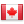• Linear Equations in Two Variables
• Word Problems

Student assignments, create unlimited student assignments., online practice, online tests, printable worksheets and tests.

## Subtract across zero word problems grade 4## Cynthia Smith

Subtract across zero word problems

• Microsoft Teams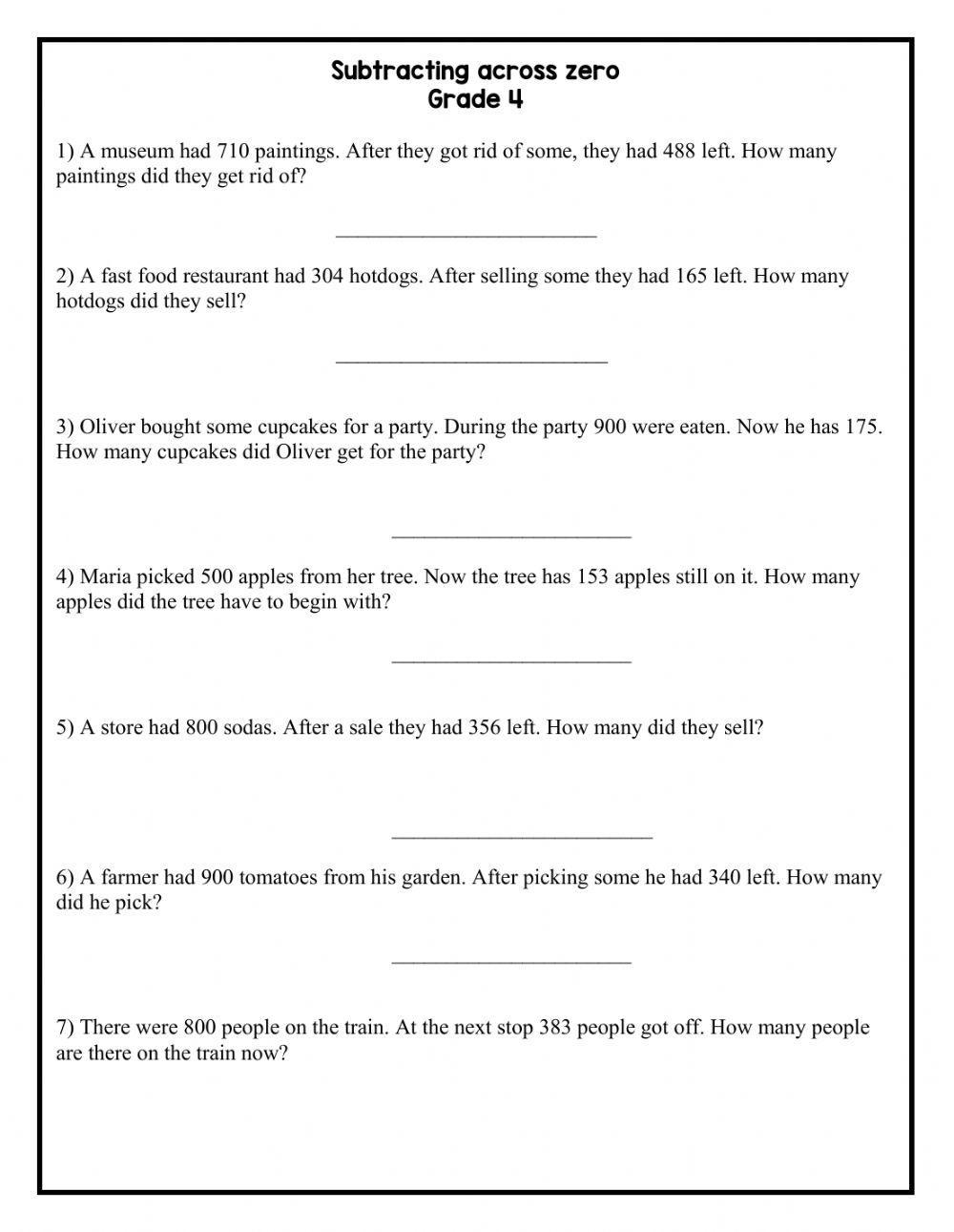• Most Popular
• Most Recent#### IMAGES2. Algebra Worksheets Grade 9 / Grade 9 Algebra Word Problems Examples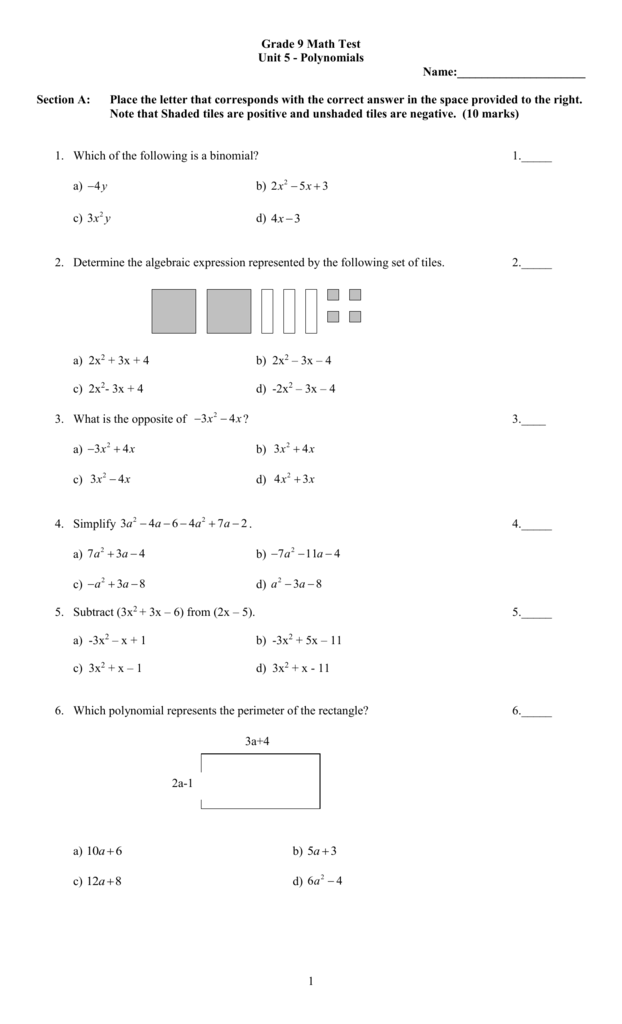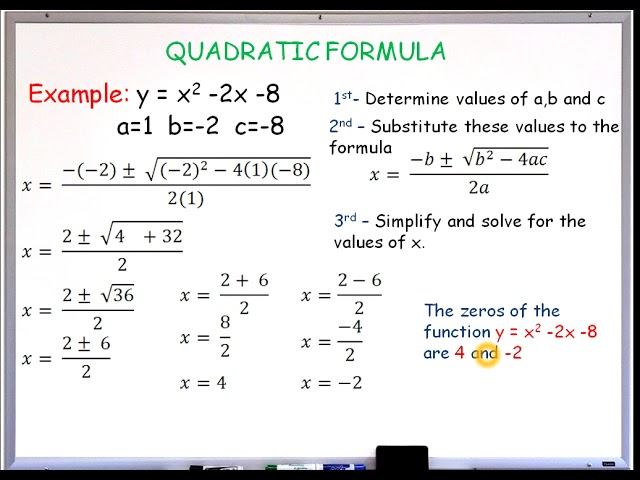4. Algebra Word Problems Worksheet / Addition Word Problems : In these6. 9th Grade Math Word Problems Worksheets Pdf#### VIDEO

1. Mathematics 2074 07 20 Word Equation

4. 📚Math challenge🤔 || #shorts #youtubeshorts #short

6. 5th & 6th Grade Word Problems Session 1

1. Grade 9 Algebra Word Problems

Example 1: A mother is three times as old as her daughter. Six years ago, the mother's age was six tines that of her daughter. How old are they now? Solution: Let x represent the daughter's age. Therefore, 3x is the mother's age. 6 (x - 6) = 3x - 6 6x - 6 = 3x - 6 3x = 30 x = 10

2. The Best Free 9th Grade Math Resources

This collection shares over 100 free printable 9th grade math worksheets on topics including order of operations, fractions and decimals, solving equations, and graphing. Plus every worksheet includes a free answer key.

3. 9th Grade Word Problems Worksheets

Mathematics Word Problems Download Add to Favorites WORKSHEETS Real Numbers, Algebra, and Problem Solving, Set 2 The word problems in this printable will give children practice with their algebra and problem solving skills. Subjects:

4. 9th Grade Word Problems Worksheets Free

Created by. Jessica Wilkerson. Common Core Math Standard: 8.F2 This worksheet gives four sets of word problems with linear situations in different representations, including verbal descriptions, graphs, tables, and equations. Students must analyze the different representations, then answer questions comparing rates of change and initial value ...

5. 9th Grade Math Worksheets: FREE & Printable

Here is a comprehensive collection of FREE printable 9th-grade Math worksheets that would help students in 9th Grade Math preparation and practice. Download our free Mathematics worksheets for 9th Grade Math. Hope you enjoy it!

6. Math Word Problem Worksheets

Grade 5 word problems worksheets. Mixed 4 operations (addition, subtraction, multiplication, division) Estimating and rounding word problems (based on the 4 operations) Add and subtract fractions and mixed numbers (like and unlike denominators) Multiplying and dividing fractions. Mixed operations with fractions (add, subtract, multiply, divide)

7. 50+ Math worksheets for 9th Grade on Quizizz

50+ Math worksheets for 9th Grade on Quizizz | Free & Printable Free Printable Math Worksheets for 9th Grade grade 9 Math Explore worksheets by Math topics The Basics Expressions and Equations Linear Functions Factoring Inequalities and System of Equations Monomials Operations Quadratic Polynomial Operations Radical Expressions Rational Expressions

8. Math Word Problems

On this page, you will find Math word and story problems worksheets with single- and multi-step solutions on a variety of math topics including addition, multiplication, subtraction, division and other math topics.

9. Free Math Worksheets

Our free math worksheets cover the full range of elementary school math skills from numbers and counting through fractions, decimals, word problems and more. All worksheets are printable files with answers on the 2nd page. Math worksheets by grade: Math worksheets by topic: Sample Math Worksheet What is K5?

10. Word Problems

Word Problems Word problems are one of the first ways we see applied math, and also one of the most anxiety producing math challenges many grade school kids face. This page has a great collection of word problems that provide a gentle introduction to word problems for all four basic math operations.

11. Math Word Problems Worksheets

Read, explore, and solve over 1000 math word problems based on addition, subtraction, multiplication, division, fraction, decimal, ratio and more. These word problems help children hone their reading and analytical skills; understand the real-life application of math operations and other math topics.

12. Free Math Worksheets

Algebra 1 High school geometry Algebra 2 Trigonometry Statistics and probability High school statistics AP®︎/College Statistics Precalculus Differential Calculus Integral Calculus AP®︎/College Calculus AB AP®︎/College Calculus BC Calculus 1 Calculus 2 Multivariable calculus Differential equations Linear algebra Early math Counting

13. 9th Grade Math Worksheets & Printables

9th Grade Math Worksheets Find quick and easy math practice for your high school freshmen with our 9th grade math worksheets. Print them to use in your classroom as part of your...

The numbers x , y , z and w have an average equal to 25. The average of x , y and z is equal to 27. Find w. Find x , y , z so that the numbers 41 , 46 , x , y , z have a mean of 50 and a mode of 45. A is a constant. Find A such that the equation 2x + 1 = 2A + 3 (x + A) has a solution at x = 2.

15. Dynamically Created Word Problems

These mixed operations word problems worksheets will produce addition, multiplication, subtraction and division problems with 1 or 2 digit numbers. These word problems worksheets will produce ten problems per worksheet. These word problems worksheets are appropriate for 3rd Grade, 4th Grade, and 5th Grade.

16. Word Problems For 9th Grade Worksheets

Displaying top 8 worksheets found for - Word Problems For 9th Grade. Some of the worksheets for this concept are Sample problems for grade 9 mathematics, Grade 9 simplifying radical expressions, Summer package pre requisite algebra skills, 9th grade math packet, 501 math word problems, Word problem practice workbook, Dear wccs students, Lake washington institute of technology lake washington.

17. Word Problems Grade 9 Worksheets

Displaying top 8 worksheets found for - Word Problems Grade 9. Some of the worksheets for this concept are Fraction word problems, Fraction multiplication word problems, Word problem practice workbook, 501 math word problems, Percent word problems, Solving proportion word problems, Two step word problems, The pythagorean theorem date period.

18. IXL

hr. min. sec. SmartScore. out of 100. IXL's SmartScore is a dynamic measure of progress towards mastery, rather than a percentage grade. It tracks your skill level as you tackle progressively more difficult questions. Consistently answer questions correctly to reach excellence (90), or conquer the Challenge Zone to achieve mastery (100)!

19. Word Problems

Word Problems - 9 Word problems ID: 1866268 Language: English School subject: Math Grade/level: Grade 1 Age: 5-7 Main content: Word problems Other contents: Add to my workbooks (0) Download file pdf Embed in my website or blog ... More Math interactive worksheets. Matching Number Name to Numbers (1-10) by khosang: Addition and subtraction 3 digit

20. Grade 9 Algebra Word Problems Worksheets

Grade 9 Algebra Word Problems - Displaying top 8 worksheets found for this concept.. Some of the worksheets for this concept are Algebra word problems no problem, Geometry word problems no problem, Word problem practice workbook, Mat 070 algebra i word problems, Finding the mean median mode practice problems, Two step word problems, 501 algebra questions 2nd edition, Solving linear equations.

21. Word Problems Grade 9 Worksheets

Word Problems Grade 9 - Displaying top 8 worksheets found for this concept.. Some of the worksheets for this concept are Fraction word problems, Fraction multiplication word problems, Word problem practice workbook, 501 math word problems, Percent word problems, Solving proportion word problems, Two step word problems, The pythagorean theorem date period.